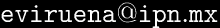# Graphics of functions f : [ax, bx] × [ay, by] → R

by Eduardo Virueña Silva

# f : [ax, bx] × [ay, by] → R

Suppose ax ≤ bx and ay ≤ by, are real numbers. Let f be a real-valued function defined on the rectangle [ax, bx] × [ay, by].

To create the graphic of function f in format .PDF fill this form:

## f : [ax, bx] × [ay, by] → R

ax bx
ay by
Nx Ny
ScaleX ScaleY ScaleZ
Obs Proj
RotZ RotY
f(x,y)=

e-mail: